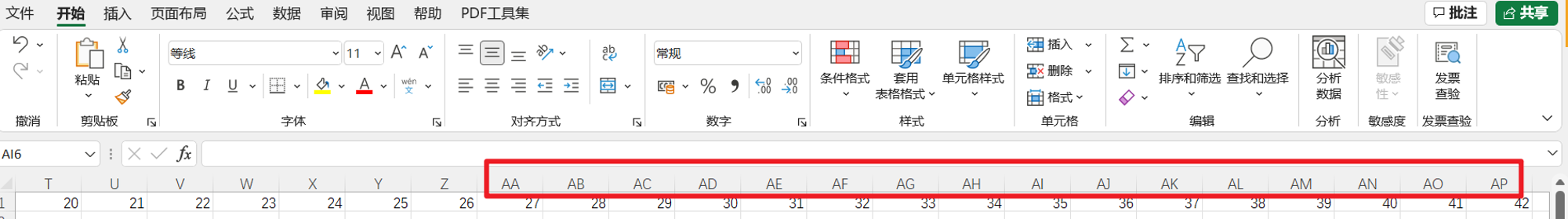AI悦创

V1

2022/01/21阅读：55主题：橙心

# 02-寻找 VIP（上）-长期招收编程一对一

1. 因为最近一对一学员有些多，所以公众号没有时间排版，这篇文章其实早已写完。
2. 在给我的一对一学员写文章，些什么文章？——Python 基础入门文章，这个系列文章我预估 28 篇，后期会考虑继续写进阶的 Python 或者结合现有的资源免费让大家看。对！免费，加我微信：Jiabcdefh，就可以免费阅读。（如果微信加不了，通过微信公众号：AI悦创，加我也可以噢！）

1. 第三点，就是整理资源。和发布在知识星球中。知识星球进去要交钱噢，主要是为了赚点钱吧。加入星球后，后期全部课程资源免费获取。（具体啥资源不言而喻）

PS：关注公众号：AI悦创，并且转发本文章：优惠价 60元（原件 365元）

# 1. 导语

Excel 是 Windows 下最流行的电子表格处理工具，在大部分公司的办公过程中，它都扮演着举足轻重的作用。年度预算、财务报表、业务订单等等，大量的商业场景中都会有 Excel 的身影。

Excel 本身支持用户通过菜单点选，进行数据处理工作。但设想这样的场景：

• 从几千行数据中挑选特殊的某些行，根据要求进行修改；
• 或者在不同表格之间进行拷贝粘贴。当由人力长期进行这样的操作时，是十分枯燥，而且极易出错的。

# 3. 课程内容

## 3.2 工作簿

Excel 文件被称为工作簿，每个工作簿中，包含一个或者多个工作表。

# 5. Excel读取

``import openpyxlwb = openpyxl.load_workbook('relayfood.xlsx')print(type(wb))# <class 'openpyxl.workbook.workbook.Workbook'>``

## 5.1 读取工作表信息

``# 获取工作簿中所有的工作表名import openpyxlwb = openpyxl.load_workbook('relayfood.xlsx')# print(type(wb))print(wb.sheetnames)# ['2009-01', '2009-02', '2009-03', '2009-04', '2009-05', '2009-06', '2009-07', '2009-08', '2009-09', '2009-10', '2009-11', '2009-12', '2010-01', '2010-02']``

## 5.2 读取单元格内容

1. 选择某个工作表。
2. 找到单元格所在的行跟列，点选。

``import openpyxlwb = openpyxl.load_workbook('relayfood.xlsx')sheet = wb['2009-04']print(type(sheet))print(sheet['B11'])print(sheet['B11'].value)``

``import openpyxlwb = openpyxl.load_workbook('relayfood.xlsx')print(wb['2009-04']['B11'].value)``

## 5.3 使用数字方式访问行列在对列进行索引的时候，Excel 默认使用字母，当列数非常多时，会出现 AA、AB 这样两个字母甚至更多的三个字母组成的列索引。比起用数字来标识列编号，用字母的方式显得麻烦很多。

openpyxl 也为我们提供了使用数字来索引列的方法。比如可以通过下面的方式读取第 11 行第 2 列（B列）的内容：

``import openpyxlwb = openpyxl.load_workbook('relayfood.xlsx')sheet = wb['2009-04']print(sheet.cell(row=11, column=2))print(sheet.cell(row=11, column=2).value)``

``import openpyxlwb = openpyxl.load_workbook('relayfood.xlsx')sheet = wb['2009-04']# TODO，使用 for 循环打印 2009-04 这个工作表中，第 10 行，第2到第4列的元素值``

``# TODO，使用for循环打印2009-04这个工作表中，第10行，第2到第4列的元素值for i in range(2, 5):    print(sheet.cell(row=10, column=i).value)``

AI悦创·推出辅导班啦，包括「Python 语言辅导班、C++辅导班、算法/数据结构辅导班、少儿编程、pygame 游戏开发」，全部都是一对一教学：一对一辅导 + 一对一答疑 + 布置作业 + 项目实践等。QQ、微信在线，随时响应！V：Jiabcdefh

## 5.4 Cell 的其它属性

``import openpyxlwb = openpyxl.load_workbook('relayfood.xlsx')print('row is ', wb['2009-04']['B11'].row)print('column is ', wb['2009-04']['B11'].column)print('coordinate is ',  wb['2009-04']['B11'].coordinate)``

• row 属性表示的就是单元格的行编号（数字）
• column 属性标识的是单元格的列编号（数字）
• 而 coordinate 表示的是类似 B11 这样的，由字母和数字组成的单元格位置坐标。

## 5.5 表的尺寸

``import openpyxlwb = openpyxl.load_workbook('relayfood.xlsx')sheet = wb['2009-04']print('行数：', sheet.max_row)print('列数：', sheet.max_column)``

``import openpyxlwb = openpyxl.load_workbook('relayfood.xlsx')sheet = wb['2009-04']# TODO，遍历 2009-04 这张工作表的每个单元格，并打印内容``

``import openpyxlwb = openpyxl.load_workbook('relayfood.xlsx')sheet = wb['2009-04']rows_num = sheet.max_rowcolumns_num = sheet.max_columnfor i in range(1, rows_num+1):    for j in range(1, columns_num+1):        print(sheet.cell(row=i, column=j).value, end=' ')    print()# 副本# 先 row 后 columnfor row in range(1, rows_num+1): for column in range(1, columns_num + 1):  print(sheet.cell(row=row, column=column).value, end=' ') print()``

## 5.6 获取行列切片

``import openpyxlwb = openpyxl.load_workbook('relayfood.xlsx')sheet = wb['2009-02']# TODO，替换下面代码中的xxx，获取红色矩形区域的内容。print(sheet['B3':xxx])``

``# TODO，替换下面代码中的xxx，获取红色矩形区域的内容。print(sheet['B3':'D7'])``

``import openpyxlwb = openpyxl.load_workbook('relayfood.xlsx')sheet = wb['2009-02']for row in sheet['B3':'D7']:    for cell in row:        print(cell.value, end=' ')    print()``

AI悦创·推出辅导班啦，包括「Python 语言辅导班、C++辅导班、算法/数据结构辅导班、少儿编程、pygame 游戏开发」，全部都是一对一教学：一对一辅导 + 一对一答疑 + 布置作业 + 项目实践等。QQ、微信在线，随时响应！V：Jiabcdefh

``import openpyxlwb = openpyxl.load_workbook('relayfood.xlsx')sheet = wb['2009-02']print(list(sheet.rows))# 补充for r in sheet.rows: print(r)# for c in sheet.columns:#  print(c)``

1. `sheet.rows` 转换成列表（list）类型。
2. 通过 `` 获取了列表的第 2 个元素（就是第 2 行）。

``import openpyxlwb = openpyxl.load_workbook('relayfood.xlsx')sheet = wb['2009-02']# TODO, 打印第4列的所有单元格内容``

``# TODO, 打印第4列的所有单元格内容column = list(sheet.columns)for cell in column:    print(cell.value)``

## 5.7 数据类型

Excel 文件单元格中的内容会有不同的类型，字符串、数字等。通过 openpyxl 读取这些内容的时候，读取到的变量在 Python 中也会拥有相应的类型。在进行计算的时候，数字可以做算术运算，而字符串不可以。类似这样的特点需要我们在处理 Excel 文件的内容时多加注意。

``import openpyxlwb = openpyxl.load_workbook('relayfood.xlsx')sheet = wb['2009-02']for cell in list(sheet.rows):    print('值：', cell.value)    print('类型：', type(cell.value))``

year 获取年
month 获取月
day 获取日

``import openpyxlwb = openpyxl.load_workbook('relayfood.xlsx')sheet = wb['2009-02']for cell in list(sheet.columns):    if cell.row > 1:        print('{}月{}日'.format(cell.value.month, cell.value.day))``

# 4. 寻找 VIP

AI悦创·推出辅导班啦，包括「Python 语言辅导班、C++辅导班、算法/数据结构辅导班、少儿编程、pygame 游戏开发」，全部都是一对一教学：一对一辅导 + 一对一答疑 + 布置作业 + 项目实践等。QQ、微信在线，随时响应！V：JiabcdefhV1